# Solution assignment 09 Quadratic equations (factorizing)

### Assignment 9

Try to solve by factorization: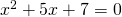### Solution

We have to find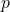and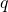such that: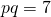and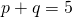There are no whole numbersandthat satisfy these equations. This does not mean that the equation has no solutions. In this case the equation has indeed no solutions because the discriminant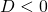(see also Quadratic equations (abc-formula)).

0